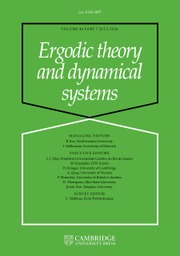Home
Hostname: page-component-dc8c957cd-p6nx7 Total loading time: 0.183 Render date: 2022-01-27T19:51:08.428Z Has data issue: true Feature Flags: { "shouldUseShareProductTool": true, "shouldUseHypothesis": true, "isUnsiloEnabled": true, "metricsAbstractViews": false, "figures": true, "newCiteModal": false, "newCitedByModal": true, "newEcommerce": true, "newUsageEvents": true }Ergodic Theory and Dynamical Systems

Abstract

Von Neumann’s original proof of the ergodic theorem is revisited. A uniform convergence rate is established under the assumption that one can control the density of the spectrum of the underlying self-adjoint operator when restricted to suitable subspaces. Explicit rates are obtained when the bound is polynomial, with applications to the linear Schrödinger and wave equations. In particular, decay estimates for time averages of solutions are shown.

MSC classification

Type
Original Article
Information
Ergodic Theory and Dynamical Systems , June 2021 , pp. 1601 - 1611

Access options

Get access to the full version of this content by using one of the access options below. (Log in options will check for institutional or personal access. Content may require purchase if you do not have access.)

References

Assani, I. and Lin, M.. On the one-sided ergodic Hilbert transform. Contemp. Math. 430 (2007), 2139.CrossRefGoogle Scholar
Ben-Artzi, M. and Klainerman, S.. Decay and regularity for the Schrödinger equation. J. Anal. Math. 58(1) (1992), 2537.CrossRefGoogle Scholar
Cycon, H. L., Froese, R. G., Kirsch, W. and Simon, B.. Schrödinger Operators with Application to Quantum Mechanics and Global Geometry. Springer, Berlin, 1987.CrossRefGoogle Scholar
Dzhulaii, N. A. and Kachurovskii, A. G.. Constants in the estimates of the rate of convergence in von Neumann’s ergodic theorem with continuous time. Sib. Math. J. 52(5) (2011), 824835.CrossRefGoogle Scholar
Evans, L. C.. Partial Differential Equations (Graduate Studies in Mathematics, 19) . American Mathematical Society, Providence, RI, 2010.Google Scholar
Kachurovskii, A. G.. The rate of convergence in ergodic theorems. Russian Math. Surveys 51(4) (1996), 653703.CrossRefGoogle Scholar
Kachurovskii, A. G.. On uniform convergence in the ergodic theorem. J. Math. Sci. 95(5) (1999), 25462551.Google Scholar
Kachurovskii, A. G.. Fejér integrals and the von Neumann ergodic theorem with continuous time. Zap. Nauchn. Sem. S.-Peterburg. Otdel. Mat. Inst. Steklov. 474 (2018), 171182.Google Scholar
Kachurovskii, A. G. and Knizhov, K. I.. Deviations of Fejer sums and rates of convergence in the von Neumann ergodic theorem. Dokl. Math. 97(3) (2018), 211214.CrossRefGoogle Scholar
Kachurovskii, A. G. and Podvigin, I. V.. Estimates of the rate of convergence in the von Neumann and Birkhoff ergodic theorems. Trans. Moscow Math. Soc. 77 (2016), 153.CrossRefGoogle Scholar
Kachurovskii, A. G. and Podvigin, I. V.. Fejér sums for periodic measures and the von Neumann ergodic theorem. Dokl. Math. 98(1) (2018), 344347.CrossRefGoogle Scholar
Kachurovskii, A. G. and Reshetenko, A. V.. On the rate of convergence in von Neumann’s ergodic theorem with continuous time. Sb. Math. 201(4) (2010), 493500.CrossRefGoogle Scholar
Kachurovskii, A. G. and Sedalishchev, V. V.. On the constants in the estimates of the rate of convergence in von Neumann’s ergodic theorem. Math. Notes 87(5–6) (2010), 720727.CrossRefGoogle Scholar
Kachurovskii, A. G. and Sedalishchev, V. V.. Constants in estimates for the rates of convergence in von Neumann’s and Birkhoff’s ergodic theorems. Sb. Math. 202(8) (2011), 11051125.CrossRefGoogle Scholar
Kato, T.. Perturbation Theory for Linear Operators. Springer, Heidelberg, 1995.CrossRefGoogle Scholar
von Neumann, J.. Proof of the quasi-ergodic hypothesis. Proc. Natl. Acad. Sci. USA 18(2) (1932), 7082.CrossRefGoogle Scholar
Riesz, F.. Some mean ergodic theorems. J. Lond. Math. Soc. (2) s1‐13(4) (1938), 274278.CrossRefGoogle Scholar
Tao, T.. Nonlinear Dispersive Equations: Local and Global Analysis (Regional Conference Series in Mathematics, 106) . American Mathematical Society/CBMS, Providence, RI, 2006.CrossRefGoogle Scholar
Tataru, D.. Strichartz estimates for second order hyperbolic operators with nonsmooth coefficients, II. Amer. J. Math. 123(3) (2001), 385423.CrossRefGoogle Scholar

Send article to Kindle

Note you can select to send to either the @free.kindle.com or @kindle.com variations. ‘@free.kindle.com’ emails are free but can only be sent to your device when it is connected to wi-fi. ‘@kindle.com’ emails can be delivered even when you are not connected to wi-fi, but note that service fees apply.

Find out more about the Kindle Personal Document Service.

Uniform convergence in von Neumann’s ergodic theorem in the absence of a spectral gap
Available formats
×

Send article to Dropbox

To send this article to your Dropbox account, please select one or more formats and confirm that you agree to abide by our usage policies. If this is the first time you use this feature, you will be asked to authorise Cambridge Core to connect with your <service> account. Find out more about sending content to Dropbox.

Uniform convergence in von Neumann’s ergodic theorem in the absence of a spectral gap
Available formats
×

To send this article to your Google Drive account, please select one or more formats and confirm that you agree to abide by our usage policies. If this is the first time you use this feature, you will be asked to authorise Cambridge Core to connect with your <service> account. Find out more about sending content to Google Drive.

Uniform convergence in von Neumann’s ergodic theorem in the absence of a spectral gap
Available formats
×
×